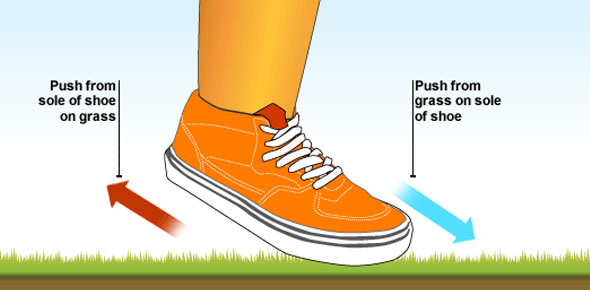How Much Do You Know About Motion And Force?

10 Questions | Total Attempts: 206SettingsThis quiz covers Chapter of the Motion Commotion book. It will help students and learners to recall and learn concepts of Motion. This quiz is intended to cover forces, motion, friction, Newton's Laws, mass and weight. Read the questions carefully. So, let's try out the quiz. All the best!

• 1.
Which of these is not a force?
• A.

Gravity

• B.

Friction

• C.

Speed

• D.

Magnetism

• 2.
All of these are needed to give a precise description of motion except:
• A.

Weight

• B.

Position

• C.

Speed

• D.

Velocity

• E.

Acceleration

• 3.
Force is measured in what units?
• A.

Joules

• B.

Meters per second

• C.

Kilometers per hour

• D.

Newtons

• 4.
An object in motion will remain in motion, and an object at rest will remain at rest until acted upon by an outside _________.
• A.

Person

• B.

Weight

• C.

Force

• D.

Mass

• 5.
The distance covered per unit of time
• A.

Kinetic

• B.

Velocity

• C.

Inertia

• D.

Speed

• 6.
Speed in a given direction
• A.

Accleration

• B.

Velocity

• C.

Momentum

• D.

Inertia

• 7.
The tendency of a moving object to keep moving
• A.

Kinetic

• B.

Gravity

• C.

Momentum

• D.

Inertia

• 8.
The rate at which velocity changes over time
• A.

Acceleration

• B.

Speed

• C.

Inertia

• D.

Kinetic

• 9.
Energy of motion
• A.

Inertia

• B.

Kinetic

• C.

Momentum

• D.

Centripetal

• 10.
The attractive force between two objects
• A.

Gravity

• B.

Speed

• C.

Mass

• D.

Newton

Related TopicsBack to top